# 1. Introduction to C

• 1.1 Unit Objectives
• 1.2 Standard C program example:
• 1.3 C Basics
• # 2. Input and Output

• 2.1 Unit Objectives
• 2.2 Initial instalation
• 2.4 The PCB board
• # 3. Program and Microcontroller

• 3.1 Code analysis
• 3.2 What is register?
• 3.3 While(1) and if(expression)
• # 4. Examples

• 4.2 Switch bounce
• 4.3 Button with action qulifier of set
• 4.4 Arduino IDE, State Machine.
• In this page you will learn the common problem using a mechanical switch and how to overcome this issue using embedded programming.

# Embedeed Programming

An embedded system contains a microcontroller to accomplish its job of processing system inputs and generating system outputs. The link between system inputs and outputs is provided by a coded algorithm stored within the processor's resident memory. What makes embedded system design so interesting and challenging is the design must also take into account the proper electrical interface for the input and output devices, limited on-chip resources, human interface concepts, the operating environment of the system, cost analysis, related standards, and manufacturing aspects. [From Barret 2010, 00/TWQ 82]

An embedded system is a computer system designed for specific control functions within a larger system, often with real-time computing constraints. It is embedded as part of a complete device often including hardware and mechanical parts. By contrast, a general-purpose computer, such as a personal computer (PC), is designed to be flexible and to meet a wide range of end-user needs. Embedded systems control many devices in common use today. Source: From Wikipedia, retrieved on 24.11.2012

# 1. Introduction to C

C programming.

## 1.1 Unit Objective

The objectives of this chapter is how to write simple C programming with input and output.

## 1.2 Standard C program example:

``` #include <avr/io.h > void main(void) { DDRD &= ~(1<<DDD2); // PD2 as Input DDRD &= ~(1<<DDD3); // PD3 as Input PORTD |= (1<<PD2); // Pullup PD2 PORTD |= (1<<PD3); // Pullup PD3 DDRB |= (1<<DDB1); // PB1 as Output while(1) { if((~PIND & (1<<PD2)) & & (~PIND &(1<<PD3))) // Button 1 and 2 pressed  PORTB |= (1<<PB1); // LED is on else  PORTB &= ~(1<<PB1); // Else LED is off } } ```

## 1.3 C Basics

Set, clear and toogle a bit

Set a bit

``` BYTE |= (1<<i); ```

Clear a bit

``` BYTE &= ~(1<<i); ```

Toogle a bit

``` BYTE ^= (1<<i); ```

# 2. Input and Output

## 2.1 Unit Objective

The objective of this unit is to write a c programming and initialize the port of attiny84 as an input and output and upload it to the

## 2.2 Initial instalation

Let's get started!

Make File

``` PROJECT=main SOURCES=\$(PROJECT).c MMCU=attiny84 MCU=t84 F_CPU = 20000000 CFLAGS=-mmcu=\$(MMCU) -Wall -Os -DF_CPU=\$(F_CPU) \$(PROJECT).hex: \$(PROJECT).out  avr-objcopy -O ihex \$(PROJECT).out \$(PROJECT).c.hex;\  avr-size --mcu=\$(MMCU) --format=avr \$(PROJECT).out \$(PROJECT).out: \$(SOURCES)  avr-gcc \$(CFLAGS) -I./ -o \$(PROJECT).out \$(SOURCES) program-bsd: \$(PROJECT).hex  avrdude -p \$(MCU) -c bsd -U flash:w:\$(PROJECT).c.hex program-dasa: \$(PROJECT).hex  avrdude -p \$(MCU) -P /dev/ttyUSB0 -c dasa -U  flash:w:\$(PROJECT).c.hex program-avrisp2: \$(PROJECT).hex  avrdude -p \$(MCU) -P usb -c avrisp2 -U  flash:w:\$(PROJECT).c.hex program-avrisp2-fuses: \$(PROJECT).hex  avrdude -p \$(MCU) -P usb -c avrisp2 -U lfuse:w:0x5E:m program-usbtiny: \$(PROJECT).hex  avrdude -p \$(MCU) -P usb -c usbtiny -U  flash:w:\$(PROJECT).c.hex program-usbtiny-fuses: \$(PROJECT).hex  avrdude -p \$(MCU) -P usb -c usbtiny -U lfuse:w:0x5E:m program-dragon: \$(PROJECT).hex  avrdude -p \$(MCU) -P usb -c dragon_isp -U  flash:w:\$(PROJECT).c.hex program-ice: \$(PROJECT).hex  avrdude -p \$(MCU) -P usb -c atmelice_isp -U  flash:w:\$(PROJECT).c.hex ```

Open and edit the make file according to your microcontroller for example for me I am using attiny 84.

main.c

``` #include <avr/io.h> int main(void){  DDRB &= ~(1<<DDB2); // PD3 as Input  DDRA |= (1<;<;DDA7); //PA7 as Output  PORTB |= (1<<PB2); //Pullup PB2 while (1) {  if ((~PINB & (1 << PB2))); //button is pressed   PORTA |= (1<<PA7); // LED is on  else   PORTA &= ~(1<<PA7); ; Else LED is off } } ```

Description:

• Switch 1 to PB2
• LED to PA7
• 1. Make makefile.

``` \$ make -f makefile.make ```

Out:2. Make fuse.

This is only done once, skip this step if you have done it.

``` \$ sudo make -f makefile.make program-usbtiny-fuses ```

Out:3. Upload the c program in the main.c file.

``` \$ sudo make -f makefile.make program-usbtiny ```

Out: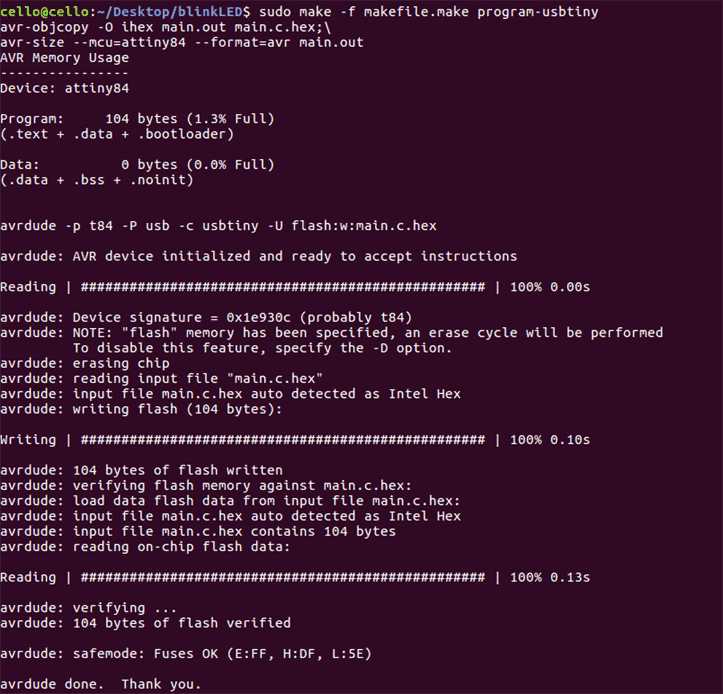This is the result if you have made it successful.

Make file

Main.c

init.c file

``` /* This file contains all init steps in one function. To call this function just use init(); */ #include <avr/io.h> #include "init.h" void init(void){ DDRD &= ~(1 < < DDD2); // set PD2 data direction to input DDRD &= ~(1 < < DDD3); // set PD data direction to input PORTD |= (1 < < PD2); // enable internal pullup for PD2 PORTD |= (1 << PD3); // enable internal pullup for PD3 DDRB |= (1 < < DDB1); // set PB1 data direction to output DDRB |= (1 < < DDB2); // set PB2 data direction to output DDRB |= (1 < < DDB3); // set PB3 data direction to output } ```

main.c file

``` #include <avr/io.h > #include "init.h" int main(void){ init (); while(1){ if ((~PIND & (1 << PD2)) && (~PIND & (1 << PD3))) { //if PD2 and PD3 are pressed  PORTB &= ~(1 << PB1); // turn led 1 off  PORTB |= (1 << PB2); // turn led 2 on  PORTB &= ~(1 << PB3); // turn led 3 off } else if ((~PIND & (1 << PD2)) || (~PIND & (1 << PD3))) {//if PD2 or PD3 is pressed  PORTB |= (1 << PB2); // turn led 2 on  PORTB |= (1 << PB3); // turn led 3 on  PORTB &= ~(1 << PB1); // turn led 1 off } else  PORTB |= (1 << PB1); // turn led 1 on  PORTB &= ~(1 << PB2); // turn led 2 off  PORTB &= ~(1 << PB3); // turn led 3 off } return 0; } ```

Description:

• Switch 1 to PD2
• Switch2 2 to PD3
• Red LED to PB1
• Green LED to PB2
• Yellow LED to PB3
• # 2.5 The PCB board

The board that I used this week is the board that I fabricated before and it is documented on the 6th exercise. It has a button and an LED.## Datasheet AT Tiny 84

I have checked the data sheet of AT Tiny 84 and this is the pinout of the microcontroller.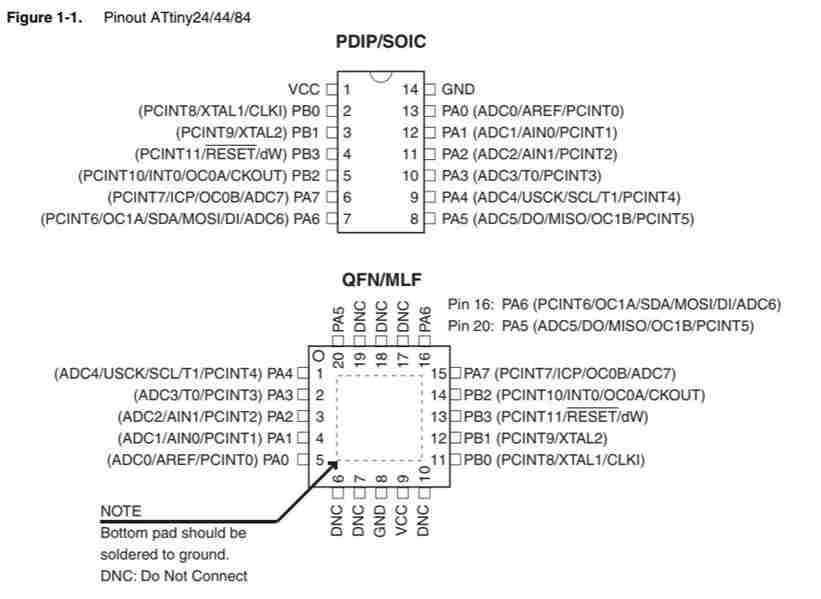# 3. Program and Microcontroller

## 3.1 Code analysis

``` void main (void){ } ```

Main is the name of the function any program must have. Program executes the main function, and other functions are used only if function main() calls them!

DDRD stands for Data Direction Register D. Notice that microcontroller has 3 groups of digital input output pins: D,B, C.

## 3.3 What is register?

It is a part of the memory the device has. But it is not just a memory for any information: every register has very specific function, which is always hinted by the name of the register.All registers in Atmega84 are 8bit long. 0000 0000 or 1111 1111,etc

``` PORTD |= (1 < < PD2); ```

The button is disconnected when it is not pressed and when it is pressed you close contact supplying low voltage to the connected pin which is called active low.

Let us define an input device of a button to pins D2.

1 < < DDD1 means to place a 1 to the position corresponding to D2 pin: DDRD = 0000 0100

~( A ) means to invert the variable A , all 0's become 1's and vice versa: 1111 1011

B & = C is a bitwise AND, which will keep the value of the bits in B, if C has a 1 at their position and make them 0 if C has 0 in their position. C is a so called mask.

The input is connected to Vdd, logical1 , throught he pullup resistor when the witch is open

Why should have 0 in the bits of DDRD corrsponding to D2 because specification specifies that zeros in DDRs are for input.

Now let set port B as an output of a LED.

Set a biwise OR |= of a a set one bit of aregister to 1 or high

More about the port operations in AVR.

## 3.3 While(1) and if(expression)

While (1) means an infinite loop and will run forever whithin the curly brackets.

If expression, if( (~PIND & (1 < < PD2)) && (~PIND & (1 < < PD3)) )

If both buttons are pressed this expression will be true and the whole expression within the brackets will be evaluated.

# 4. Examples

main.c

``` #include <avr/io.h> int main(void){  DDRB &= ~(1<<DDB2); // PD3 as Input  DDRA |= (1<;<;DDA7); //PA7 as Output  PORTB |= (1<<PB2); //Pullup PB2 while (1) {  if ((~PINB & (1 << PB2))); //button is pressed   PORTA |= (1<<PA7); // LED is on  else   PORTA &= ~(1<<PA7); ; Else LED is off _delay_ms(1000); } } ```

Description:

width="320" height="240"
• Switch 1 to PB2
• LED to PA7

click progarm c

make file

# 4.2 Switch bounce

We have almost finished with inputs but there is one unpleasant feature that you need to be aware of switch bounce. The contacts of a switch are mechanical. When the switch is operated they do not open or close cleanly but vibrate for a while. This causes the contacts to open and close several times, each time the switch is operated.Input signal with 'bounce'. This typically lasts for around 50 ms. It can be eliminated by using extra components or (more usually) in software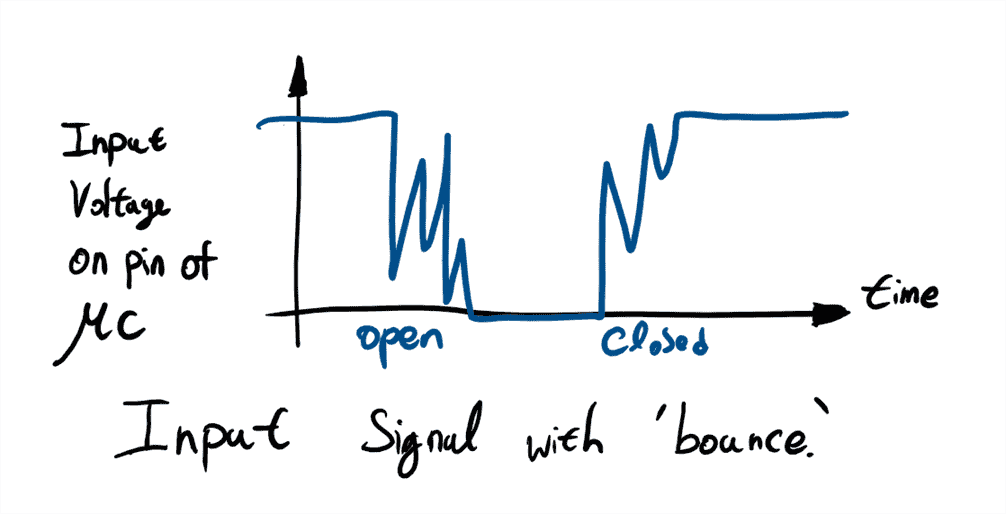Debouncing

Problem: Buttons, as well as switches described on previous slide, are prone to signal spikes after the press. It can result in many readings per one actual click. The possible hardware solution would be RC circuit: Here the signal noise, which has high frequency, is filtered by capacitor. However, it would be a lot easier if we can solve the problem in the software!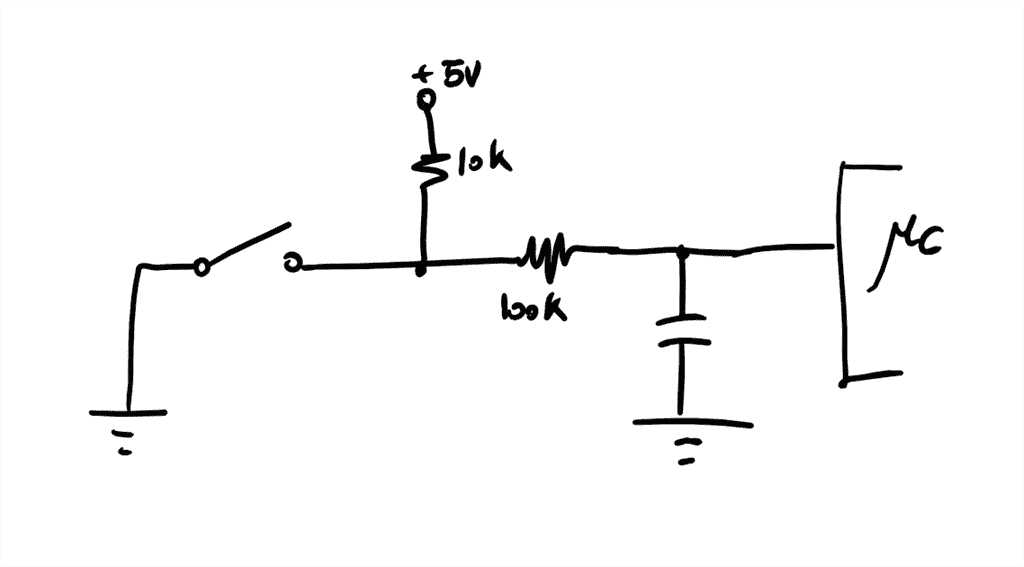## 4.3 Button with action qulifier of set

In this exercise we will have one input and one output. The LED as an output will turn on once the button is clicked and to turn off the LED once the button has to be clicked again. To program this, we will use a state space machine.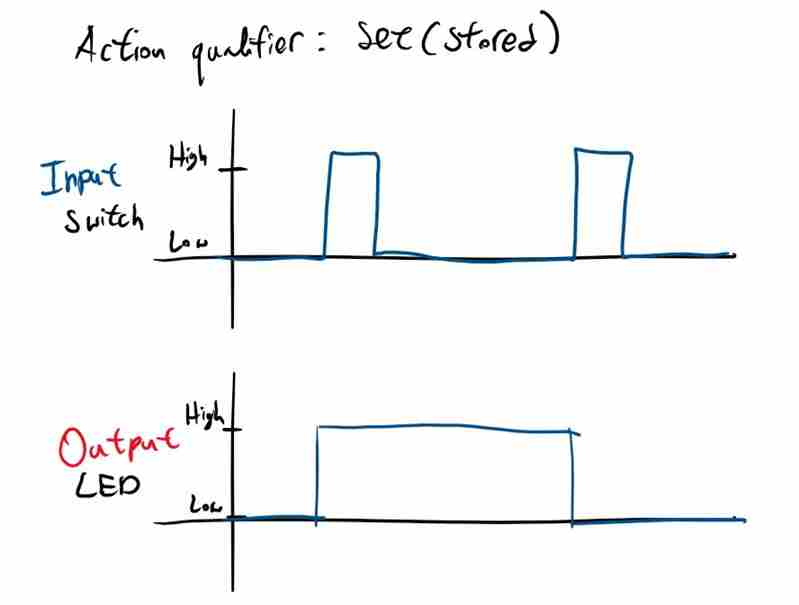main.c

``` #include <avr/io.h> #include <util/delay.h> void init(void){  //set inputs and outputs  DDRB &= ~(1<<DDB2); // PD3 as Input  DDRA |= (1<<DDA7); // PA7 as Output  PORTB |= (1<<PB2); // Pullup PB2 } int main(void){  //state  int state=0; //starting with state 0 and wait for the button to be pressed  int debounceTicks = 50; // delay of millisec that have to pass by before a click and it is assumed to be safe while (1) {  //starting with state 0 i f (state ==0) { // waiting a button to be pressed   if ((~PINB & (1 << PB2))){ //button is pressed    _delay_ms(debounceTicks);    state =1;   } }else if(state ==1){  _delay_ms(debounceTicks);   if ((PINB & (1 << PB2))){ //button is not pressed    state=2;   } }else if(state ==2){  click();  state =0; } ```

Video

State machine diagaram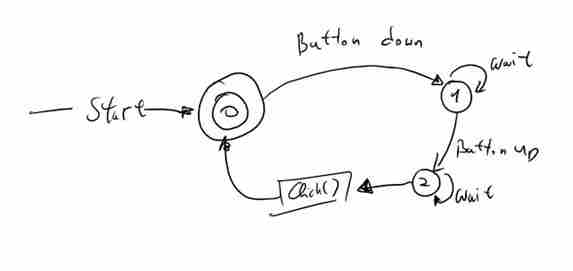click progarm c

make file

## 4.4 Arduino IDE, Button program for click, double clicks and long press.

I used an arduino IDE to program this using a state machine.

The advantages of using arduino IDE is that it has a core set of library functions that control the peripheral hardware of whatever microcontroller you are using. In pure C, you need the datasheet for the specific microcontroller your are using. It requires more work, more reading, more coding and more debugging but the resulting progam will probably be smaller and more efficient.

In this chapter, we are going to program to do something when the buton is click, double clicks and long pressed.

The board that I used is modified from Satshakit-128. I have added one button and a LED.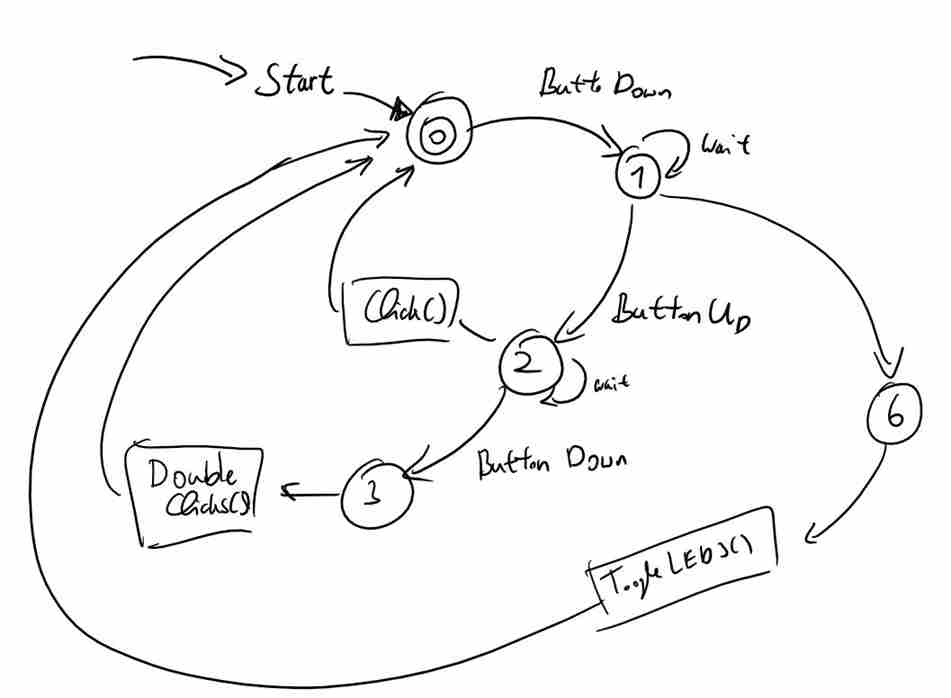The LED 1 will toogle once it is click, the LED 2 will toogle once the button is double clicked and if the button is pressed in long term, LED 3 will turn on for one second.

Above is the code for having click, double clicks and long pressed using a state space machine.

``` // Input pin int button =0; // select the pin for the button // Output pin int ledPinYes = 13; // select the pin for the YES LED change the click function, pin 13 int ledPinNo = 12; // select the pin for the No LED change the double click function, pin 12 int ledPinNope = 11; // select the pin for the Nope LED pin 11 // Input Values boolean buttonState = false; // choose the input pin (for a pushbutton) unsigned long now = millis(); // current (relative) time in msecs. //State int _state = 0; // starting with state 0: waiting for button to be pressed unsigned long _startTime; // will be set in state 1 unsigned long _stopTime; // will be set in state 1 int _debounceTicks = 50; // number of millisec that have to pass by before a click is assumed as safe. int _clickTicks = 300; // number of millisec that have to pass by before a click is detected. int _pressTicks = 2000; // number of millisec that have to pass by before a long button press is detected. //led state to toogle boolean stateLedYes; boolean stateLedNo; void setup() { // put your setup code here, to run once: pinMode(ledPinYes, OUTPUT); // declare the ledPin as an OUTPUT pinMode(ledPinNo, OUTPUT); // declare the ledPin as an OUTPUT pinMode(ledPinNope, OUTPUT); // declare the ledPin as an OUTPUT pinMode(button, INPUT); // declare the button as an INPUT Serial.begin(9600); } void loop() { // put your main code here, to run repeatedly: buttonState = digitalRead(button); // read input value now= millis(); // update variable now //Starting (State 0) if (_state == 0) { // waiting for One pin being pressed. if ( buttonState == false) { //if button is pressed _state = 1; // step to state 1 _startTime = now; // remember starting time Serial.println("state 0!"); } }else if(_state == 1) { // waiting for One pin being released. Serial.println("state 1!"); Serial.print("Start time:"); Serial.println(_startTime); Serial.print("now:"); Serial.println(now); if ((buttonState == false) && (now > _startTime + _pressTicks)) { // /if button is pressed and pressed too long _state = 6; // step to state 6 }else if(buttonState == true) { // if button is not pressed delay(_debounceTicks); _state = 2; _stopTime = now; // remember stopping time }else if((buttonState == true) && ((unsigned long)(now - _startTime) < _debounceTicks)){ Serial.println("State1 Debouncing"); // button was released to fast means that this is debouncing _state =0; }else{ //wait. Stay in this state } }else if(_state == 6) { // waiting for One pin being release after long press. toogleNopeLED(); //Serial.println("state 6!"); if (buttonState == true) { // button is not pressed _state = 0; // restart. //Serial.println("state 6!released"); } }else if(_state ==2){ if (now > _startTime + _clickTicks ) { // this was only a single short click //Serial.println("state 2!"); click(); //toggle LED yes LED _state = 0; // restart. }else if ((buttonState == false)&& ((unsigned long)(now - _stopTime) > _debounceTicks) ){ _startTime = now; // remember starting time _state =3; } }else if(_state ==3){ if( buttonState == true){// button release and this is double click doubleClick(); Serial.println("state 3!"); //Serial.println("Double Click!"); _startTime = now; // remember starting time delay(_debounceTicks); //debouncing delay _state = 0; // restart. }else if((buttonState == true) && ((unsigned long)(now - _startTime) < _debounceTicks)){ // button was released to fast means that this is debouncing _state =0; } } } void click(void){ //PORTD ^= (1<<ledPinYes); //toogle led yes digitalWrite(ledPinYes, (stateLedYes) ? HIGH : LOW); stateLedYes = !stateLedYes; delay(100); Serial.println("Click!"); } void doubleClick(void){ digitalWrite(ledPinNo, (stateLedNo) ? HIGH : LOW); stateLedNo = !stateLedNo; delay(100); Serial.println("Double Click!"); } void toogleNopeLED(void){ digitalWrite(ledPinNope, HIGH); // turn LED ON delay(1000); digitalWrite(ledPinNope, LOW); // turn LED ON } ```

The board that I used in this video is the satshakit-128 board that I milled in the fabLab.